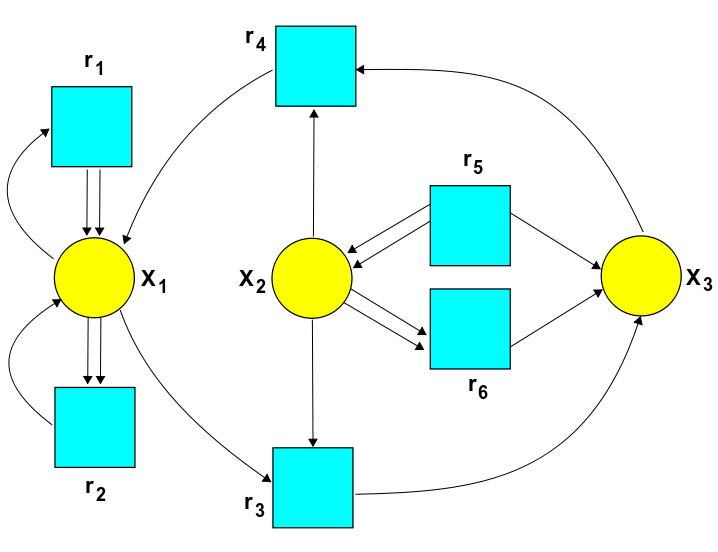The Azimuth Project Experiments in trimolecular reactions

A three concentration example

For the general terms and definitions used in this project see

Here we will consider a trimolecular reaction from Feinberg page 2253.

Equation 6.7 gives the following chemical reaction network.

$r_1: A_1 \rightarrow 2 A_1$
$r_2: 2 A_1 \rightarrow A_1$
$r_3: A_1 + A_2 \rightarrow A_3$
$r_4: A_3 \rightarrow A_1 + A_2$
$r_5: A_3 \rightarrow 2 A_2$
$r_6: 2 A_2 \rightarrow A_3$

The stochastic Petri netThe rate equation

$\frac{d}{d t} X_1 = r_1 X_1 - r_3 X_1 X_2 - r_2 X_1^2 + r_4 X_3$
$\frac{d}{d t} X_2 = -r_3 X_1 X_2 + (r_4+2 r_5) X_3 - 2 r_6 X_2^2$
$\frac{d}{d t} X_3 = r_3 X_1 X_2 + r_6 X_2^2 - (r_4+r_5) X_3$

The solutions of this equation are given as.

$X_1 = \frac{r_1}{r_2}$
$X_2 = \frac{r_1 r_3 r_5}{r_2 r_4 r_6}$
$X_3 = \frac{r_1^2 r_3^2 r_5}{r_2^2 r_4^2 r_6}$

These concentration levels make the rate equations zero for all time.

The master equation

$H = r_1(a_1^\dagger a_1^\dagger a_1 - a_1^\dagger a_1) + r_2(a_1^\dagger a_1 a_1 - a_1^\dagger a_1^\dagger a_1 a_1)+ r_3(a_3^\dagger a_1 a_2 - a_1^\dagger a_2^\dagger a_1 a_2) + r_4(a_1^\dagger a_2^\dagger a_3 - a_3^\dagger a_3) + r_5(a_2^\dagger a_2^\dagger a_3 - a_3^\dagger a_3) + r_6(a_3^\dagger a_2 a_2 - a_2^\dagger a_2^\dagger a_2 a_2)$

We let this Hamiltonian act on $\Psi := e^{c_1 z_1}e^{c_2 z_2}e^{c_3 z_3}$ and to satisfy $H\Psi = 0$ there must exist a choice of $c_1$, $c_2$ and $c_3$ that will cause all the following equations to vanish together.

$z_1; 0 = -r_1c_1 + r_2 c_1^2$
$z_1^2; 0 = r_1 c_1 - r_2 c_1^2$
$z_3; 0 = r_3 c_1 c_2 - (r_4 +r_5) c_3 + r_6 c_2^2$
$z_2^2; 0 = -r_6 c_2^2 + r_5 c_3$
$z_1 z_2; 0 = r_4 c_3 - r_3 c_1 c_2$

By picking $c_1 = X_1$, $c_2 = X_2$ and $c_3 = X_3$. This example has the following Petri net.